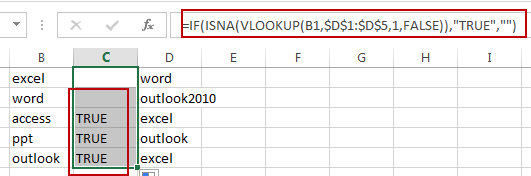# Find Unique Values in Two Columns

This post will guide you how to find unique values in two columns in Excel. How do I find unique values between two columns with a formula in Excel. How to display only unique values from two columns in Excel.

## Find Unique Values

Assuming that you have a list of data in two different columns and you want to find all unique values in two given columns. If the value is in one column but not in another column, then marked as TRUE, otherwise, marked as FALSE. How to achieve it. You can use a formula based on the IF function, the ISNA function and the VLOOKUP function.

=IF(ISNA(VLOOKUP(B1,\$D\$1:\$D\$5,1,FALSE)),”TRUE”,””)

Type this formula into the formula box of the cell C1, and then press Enter key, and drag the AutoFill Handle over other cells to apply this formula.You will see that all unique values in two different columns B have been displayed as TRUE.

### Related Functions

• Excel IF function
The Excel IF function perform a logical test to return one value if the condition is TRUE and return another value if the condition is FALSE. The IF function is a build-in function in Microsoft Excel and it is categorized as a Logical Function.The syntax of the IF function is as below:= IF (condition, [true_value], [false_value])….
• Excel VLOOKUP function
The Excel VLOOKUP function lookup a value in the first column of the table and return the value in the same row based on index_num position.The syntax of the VLOOKUP function is as below:= VLOOKUP (lookup_value, table_array, column_index_num,[range_lookup])….
• Excel ISNA function
The Excel ISNA function used to check if a cell contains the #N/A error, if so, returns TRUE; otherwise, the ISNA function returns FALSE.The syntax of the ISNA function is as below:=ISNA(value)….